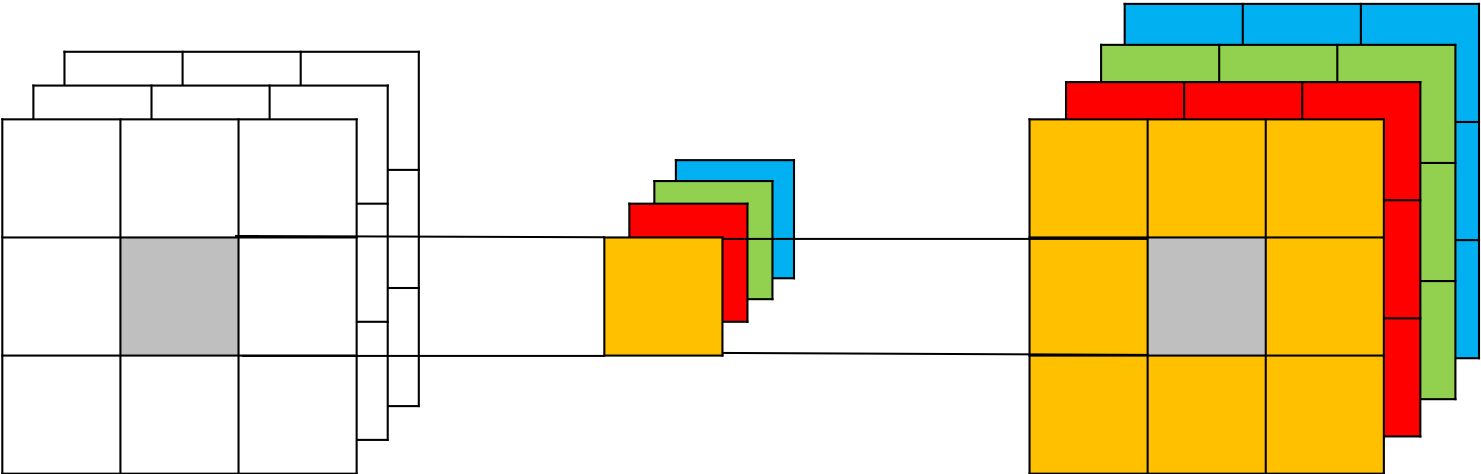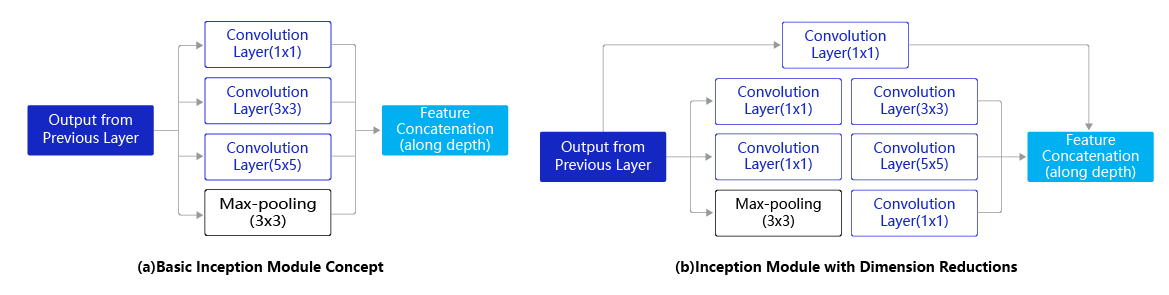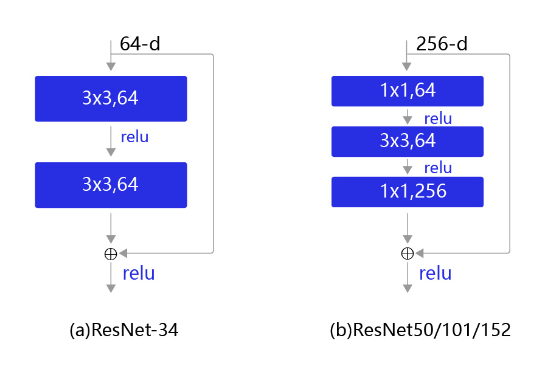# $$1\times{1}$$ 卷积（$$1\times{1}$$ Convolution）¶

## 一、1*1 卷积¶

$$1\times{1}$$ 卷积，与标准卷积完全一样，唯一的特殊点在于卷积核的尺寸是$$1\times{1}$$ ，也就是不去考虑输入数据局部信息之间的关系，而把关注点放在不同通道间。当输入矩阵的尺寸为$$3\times{3}$$ ，通道数也为3时，使用4个$$1\times{1}$$卷积核进行卷积计算，最终就会得到与输入矩阵尺寸相同，通道数为4的输出矩阵，如 图1 所示。• $$1\times{1}$$ 卷积的作用

1. 实现信息的跨通道交互与整合。考虑到卷积运算的输入输出都是3个维度（宽、高、多通道），所以$$1\times{1}$$ 卷积实际上就是对每个像素点，在不同的通道上进行线性组合，从而整合不同通道的信息。

2. 对卷积核通道数进行降维和升维，减少参数量。经过$$1\times{1}$$ 卷积后的输出保留了输入数据的原有平面结构，通过调控通道数，从而完成升维或降维的作用。

3. 利用$$1\times{1}$$ 卷积后的非线性激活函数，在保持特征图尺寸不变的前提下，大幅增加非线性

## 二、应用示例¶

• $$1\times{1}$$ 卷积在GoogLeNet中的应用Inception模块的设计思想采用多通路(multi-path)的设计形式，每个支路使用不同大小的卷积核，最终输出特征图的通道数是每个支路输出通道数的总和。如 图2(a) 所示，Inception模块使用3个不同大小的卷积核对输入图片进行卷积操作，并附加最大池化，将这4个操作的输出沿着通道维度进行拼接，构成的输出特征图将会包含经过不同大小的卷积核提取出来的特征，从而达到捕捉不同尺度信息的效果。然而，这将会导致输出通道数变得很大，尤其是将多个Inception模块串联操作的时候，模型参数量会变得非常大。

$1\times1\times192\times64+3\times3\times192\times128+5\times5\times192\times32=387072$

$\begin{split} \begin{eqnarray}\small{ 1\times1\times192\times64+1\times1\times192\times96+1\times1\times192\times16+3\times3\times96\times128+5\times5\times16\times32 \\ +1\times1\times192\times32 =163328} \end{eqnarray} \end{split}$

Inception模块的具体实现如下代码所示：

# GoogLeNet模型代码
import numpy as np
## 组网

# 定义Inception块
def __init__(self, c0, c1, c2, c3, c4, **kwargs):
'''
Inception模块的实现代码，

c1,图(b)中第一条支路1x1卷积的输出通道数，数据类型是整数
c2,图(b)中第二条支路卷积的输出通道数，数据类型是tuple或list,
其中c2是1x1卷积的输出通道数，c2是3x3
c3,图(b)中第三条支路卷积的输出通道数，数据类型是tuple或list,
其中c3是1x1卷积的输出通道数，c3是3x3
c4,图(b)中第一条支路1x1卷积的输出通道数，数据类型是整数
'''
super(Inception, self).__init__()
# 依次创建Inception块每条支路上使用到的操作
self.p1_1 = Conv2D(in_channels=c0,out_channels=c1, kernel_size=1)
self.p2_1 = Conv2D(in_channels=c0,out_channels=c2, kernel_size=1)
self.p3_1 = Conv2D(in_channels=c0,out_channels=c3, kernel_size=1)
self.p4_2 = Conv2D(in_channels=c0,out_channels=c4, kernel_size=1)

def forward(self, x):
# 支路1只包含一个1x1卷积
p1 = F.relu(self.p1_1(x))
# 支路2包含 1x1卷积 + 3x3卷积
p2 = F.relu(self.p2_2(F.relu(self.p2_1(x))))
# 支路3包含 1x1卷积 + 5x5卷积
p3 = F.relu(self.p3_2(F.relu(self.p3_1(x))))
# 支路4包含 最大池化和1x1卷积
p4 = F.relu(self.p4_2(self.p4_1(x)))
# 将每个支路的输出特征图拼接在一起作为最终的输出结果
return paddle.concat([p1, p2, p3, p4], axis=1)

• $$1\times{1}$$ 卷积在ResNet中的应用

$3\times3\times256\times256\times2=1179648$

$1\times1\times256\times64+3\times3\times64\times64+1\times1\times64\times256=69632$# ResNet模型代码
import numpy as np

# ResNet中使用了BatchNorm层，在卷积层的后面加上BatchNorm以提升数值稳定性
# 定义卷积批归一化块
def __init__(self,
num_channels,
num_filters,
filter_size,
stride=1,
groups=1,
act=None):

"""
num_channels, 卷积层的输入通道数
num_filters, 卷积层的输出通道数
stride, 卷积层的步幅
groups, 分组卷积的组数，默认groups=1不使用分组卷积
"""
super(ConvBNLayer, self).__init__()

# 创建卷积层
self._conv = nn.Conv2D(
in_channels=num_channels,
out_channels=num_filters,
kernel_size=filter_size,
stride=stride,
groups=groups,
bias_attr=False)

# 创建BatchNorm层

self.act = act

def forward(self, inputs):
y = self._conv(inputs)
y = self._batch_norm(y)
if self.act == 'leaky':
y = F.leaky_relu(x=y, negative_slope=0.1)
elif self.act == 'relu':
y = F.relu(x=y)
return y

# 定义残差块
# 每个残差块会对输入图片做三次卷积，然后跟输入图片进行短接
# 如果残差块中第三次卷积输出特征图的形状与输入不一致，则对输入图片做1x1卷积，将其输出形状调整成一致
def __init__(self,
num_channels,
num_filters,
stride,
shortcut=True):
super(BottleneckBlock, self).__init__()
# 创建第一个卷积层 1x1
self.conv0 = ConvBNLayer(
num_channels=num_channels,
num_filters=num_filters,
filter_size=1,
act='relu')
# 创建第二个卷积层 3x3
self.conv1 = ConvBNLayer(
num_channels=num_filters,
num_filters=num_filters,
filter_size=3,
stride=stride,
act='relu')
# 创建第三个卷积 1x1，但输出通道数乘以4
self.conv2 = ConvBNLayer(
num_channels=num_filters,
num_filters=num_filters * 4,
filter_size=1,
act=None)

# 如果conv2的输出跟此残差块的输入数据形状一致，则shortcut=True
# 否则shortcut = False，添加1个1x1的卷积作用在输入数据上，使其形状变成跟conv2一致
if not shortcut:
self.short = ConvBNLayer(
num_channels=num_channels,
num_filters=num_filters * 4,
filter_size=1,
stride=stride)

self.shortcut = shortcut

self._num_channels_out = num_filters * 4

def forward(self, inputs):
y = self.conv0(inputs)
conv1 = self.conv1(y)
conv2 = self.conv2(conv1)

# 如果shortcut=True，直接将inputs跟conv2的输出相加
# 否则需要对inputs进行一次卷积，将形状调整成跟conv2输出一致
if self.shortcut:
short = inputs
else:
short = self.short(inputs)